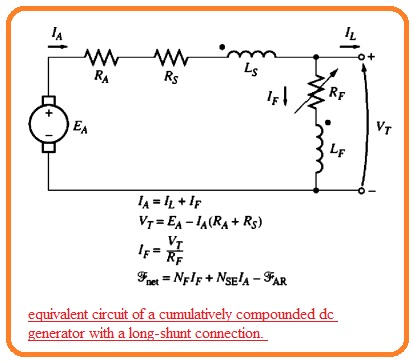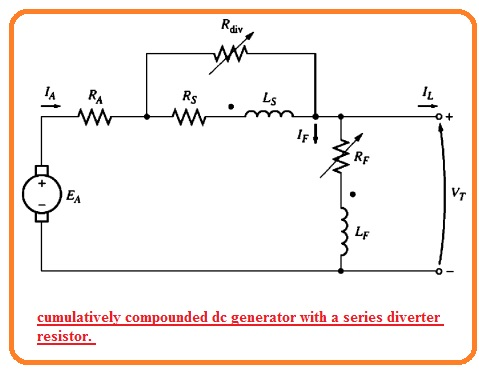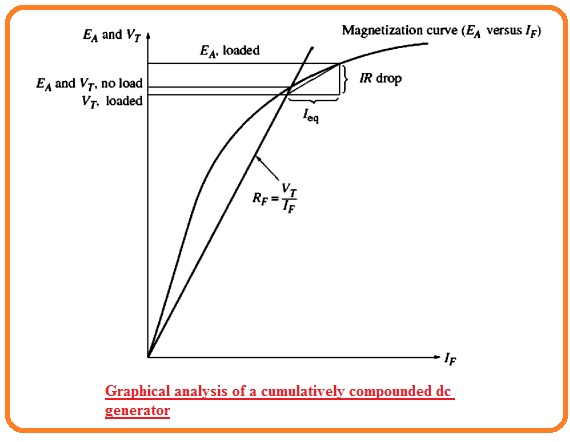Hi, reader welcome to the new post. In this post, we will have a detailed look at the Introduction to Cumulatively Compounded Dc Generator. DC generator is an electrical machine that is used to convert mechanical power into DC electrical power. There are numerous categories of dc generators such as dc series generators, dc shunt generators, and dc wound generators.

All generators produce dc power but the difference is that their structure is a combination of their armature and field windings. These connection define their names. In this post, we will discuss the working, operation application, and related parameters of a cumulatively compound dc generator. So let’s get started with Introduction to Cumulatively Compounded Dc Generator.

## Introduction to Cumulatively Compounded DC Generator

• The cumulatively compounded dc generator has such a combination of series and field windings that their net produced flux will be added to each other.
• In the below figure the equivalent circuit of this generator can be seen that has a long shunned configuration.• The dot shown at 2 field windings is used to show the direction of the current that is towards it is positive. In simple words, the current moving to the dot indicates the positive value of MMF.
• Note that the armature current moving to the dotted point of series field winding and the shunt current IF move to the dotted point of shunt field windings.
• So the net MMF for this equation is given here.

Fnet=FF+FSE-FAR

• Here FF denotes the shunt field MMF FSE indicates the series field MMF and FAR denotes the MMF due to armature reaction.
• The equivalent effective shunt field current for this generator is mentioned here.

NFl’F = NFIF + NSEIA – FAR

I’F=IF+NSE/NF xIA-FAR/NF

• The equation of current and voltage for this generator is mentioned here.

IA=IF+IL

VT=EA-IA(RA+RS)

IF=VT/RF

• There is a second connection linking of the cumulatively compound generator. That is a short shunt connection here the series field is at the outer side of the shunt field circuitry and IL is passing through this coil in place off IA.### Terminal Characteristics of Cumulatively Compounded DC Generator

• To discuss the terminal characteristic of cumulatively compound dc generators it is compulsory to study the competing effect which exists in the generator.
• Let us assume that the load at the generator rises. With the load, increment loads current IL increases.
• As IA=IF+IL the armature current also increases. At this stage, there are to factors that exist in the generator.
• AS current IA increases in results IA(RA+Rs ) losses also increase.  That results in a decrement in VT.

VT = EA – IA (RA + Rs)

• With the IA increment, the series field magnetomotive force FSE=NSEIA rises also. It raises the net MMF that is Ftot=NFIF+NSEIA that causes an increment influx in the generator.
• Due to flux increment, internally generated voltage also increases that in results VT=EA-IA(RA+RS) increases.
• these 2 factors repel one another one causes to increment in VT and the other decreases the VT.
• SO here question arises which effect dominates in the generator?
• The answer of this question is that number of turns in series to poles define the effect.
• There are different cases that also explain this question.
1. Some turn NSE: if there is less value of turns in series the resistive voltage loss effect wins hand down. The voltage decreases like in a shunt generator.• This category of assembly where full load VT is less than the no-load Vt is known as undercounted

Large Series Turns:

• The second case is that if we add some more turned-in series to the poles then ist flux strengthening effect dominates and Vt increases with load.
• Though with the load increment magnetic saturation adjusts in and resistive losses become strengthened than the flux increase factor.
• In this generator, VT firstly rises and after that decreases with the load increment.
• If the terminal voltage for no-load has the same value as the full load then a generator is known as flat compounded.

• If even further series turns are connected with the generator the flux strengthening factor nominates for long time interval till then resistive losses dominate.
• This results in the characteristic having full load VT larger than the no-load VT.
• If the terminal voltage for full loaded condition is larger than VT for no-load the generator state is known as over compounded.

This condition can see in the below figure.• We can also discuss all these voltage characteristics for one generator in use of diverter resistance.
• In below figure you can see the generator having a large number of series turns NSE.
• The diverter resistance is linkked to the ssereis field.
• If resistance Rdh is set to a larger value mostly part of IA will pass in the series field windings and the generator is overcompounded.
• If we change the value of resistance Rdiv to less value then a larger part of the current about series field windings passes through Rdiv this state of the generator called uncompounded.### Voltage Control of Cumulatively Compounded DC Generators

• The methods used for voltage control of cumulatively compound dc generators are similar to those used for shunt dc generators.
• Vary the rotation speed. The increment in W results in increases in internal generated voltage EA=KφW that causes the increment in terminal voltage.
• Vary Field current: The decrement in RF results increment in field current that rises the net MFF of the generator. With the Ftot rises flux in the generator increases and internally generated voltage increases. That results in an increment in Internal generated voltage.

### Analysis of Cumulatively Compounded DC Generators

• The below-written equations A and B are the main description of the terminal characteristics of cumulatively compounded dc generators.
• The equivalent shunt field current Ieq due to series field and armature reaction is mention here.

Ieq=NSE/NF. IA – FAR/NF

• So the value of the effective shunt field current in generator is given here.

IF’=IF+Ieq

• The equivalent current Ieq denotes the horizontal distance at the left and right sides of FR with the axes of the magnetization curve.
• The resistance losses in the generator is mentioned here through the IA(RA+Rs) that strength relies at the IA.
• So they make 2 sides triangle that has a magnitude function of IA.
• To determine the output voltage for the respected load find the size of the triangle and determine the point where it places among the IF and magnetization curve.
• This is explained here.• the terminal voltage for no-load states will be point over the resistance line and the magnetization curve meets.
• When load is linked to the generator the series field MMD rises increasing the equivalent shunt Ieq and resistive voltage losses IA(RA+Rs) in the generator.
• To determine the new output voltage in the generator move the left corner of the resulting triangle with the shunt field current line till the top point of the triangle meets the magnetization curve.
• The upper point of the triangle then denotes the internal generated voltage in a generator while the lower line denotes the VT of a generator.
• The below diagram denotes the procedures repeated numerous times to make a complete terminal characteristic for the generator.### Over Compounded Generator vs Flat Compounded Generator vs Under Compounded Generator

Feature Over Compounded Flat Compounded Under Compounded
Series field winding More turns than flat compounded Less turns than flat compounded Fewer turns than under compounded
Effect on terminal voltage Increases with load current Remains constant with load current Decreases with load current
Applications Arc welding, traction Lighting, power supply Constant speed drives

## Cumulatively Compounded vs differentially Compounded Generator

Feature Cumulatively Compounded Differentially Compounded
Series field winding Increases in turns with distance from the armature Red in turns with distance from the armature
Effect on terminal voltage Increases with load current Reduces with load current
Applications Traction, arc welding Constant speed drives

## External Characteristics of DC Compound Generator

The external characteristic of a DC compound generator is a graphical relation between terminal voltage and load current. It is a graph of terminal voltage for load current. The external characteristic of a dc compounded generator is nonlinear and defined in three types

• Over-compounded: The terminal voltage increases increment in load current. This generator is used in applications where a constant output voltage is needed also with changing load current
• Flat-compounded: The terminal voltage remains constant with load current increment.. This generator is used where constant output voltage is needed, but the load current can change widely.
• Under-compounded: The terminal voltage reduces with increasing load current. This generator is used where a high output voltage is needed at low load currents, but the terminal voltage can reduce as the load current increases

## Compounded Dc Motor vs Compounded Dc Generator

Feature Compounded DC Motor Compounded DC Generator
Field windings Two windings, series, and shunt it also has the Two Windings series and Shunt
Connection of field windings Series winding is connected in series combination with the armature winding, and the shunt winding is connected in parallel combination with the armature winding Series winding is attached in series with the armature winding, and the shunt winding is configured in parallel with the armature winding
Torque-speed characteristics The torque increases with the increase in load current, but the speed is reduced slightly The terminal voltage increases with the rise in load current, but the speed minimizes slightly
Applications Used in applications where a high starting torque is needed, like hoists, cranes, and elevators Used in applications where a constant output voltage is needed, like power supplies, battery chargers, and welding machines

### Faqs

• What is a cumulatively compounded DC generator?

A cumulatively compounded DC generator is DC generator that comes with shunt filed winding and series field winding. The series winding is attached in series combination with the armature. The magnetic fluxes generated by 2 windings are additive which means that the overall magnetic field of the generator increases when the load increases

• What is the difference between cumulative and differential compound DC generators?

The basic difference between cumulative and differential compound DC generators is the technique in which the series field winding affects the overall magnetic field strength of the generator. In a cumulatively compounded generator, the series field winding is added to the magnetic field generated by the shunt field winding. In a differential compound generator, the series field winding opposes the magnetic field generated by the shunt field winding.

• What is a cumulative compound DC motor used for?

Cumulative compound DC motors are used in different applications like

• Lighting: Cumulative compound DC motors are mostly used to power DC lighting systems.
• Power supply: Cumulative compound DC motors are used to power DC power supplies.
• Heavy power services: Cumulative compound DC motors are employed in heavy power services, like traction motors for trains and elevators.
• What is the main characteristic of a cumulative compound generator?

The main characteristic of a cumulative compound generator is that the output voltage increases when the load increases. This is because the series field winding is added to the magnetic field generated by the shunt field winding, which in turn rises the output voltage.

That is a detailed post about Cumulatively Compounded Dc Generator. If you have any further query ask in the comments Thanks for reading. Have a nice day.